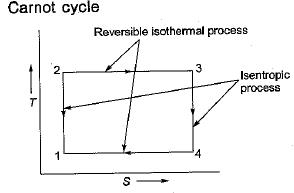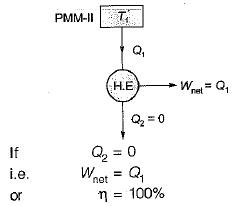Courses

# Second Law Of Thermodynamics - 2

## 10 Questions MCQ Test Thermodynamics | Second Law Of Thermodynamics - 2

Description
This mock test of Second Law Of Thermodynamics - 2 for Mechanical Engineering helps you for every Mechanical Engineering entrance exam. This contains 10 Multiple Choice Questions for Mechanical Engineering Second Law Of Thermodynamics - 2 (mcq) to study with solutions a complete question bank. The solved questions answers in this Second Law Of Thermodynamics - 2 quiz give you a good mix of easy questions and tough questions. Mechanical Engineering students definitely take this Second Law Of Thermodynamics - 2 exercise for a better result in the exam. You can find other Second Law Of Thermodynamics - 2 extra questions, long questions & short questions for Mechanical Engineering on EduRev as well by searching above.
QUESTION: 1

Solution:
QUESTION: 2

Solution:
QUESTION: 3

### The four processes of the Carnot cycle are

Solution:Hence Carnot cycle consists of two reversible isothermal and two isentropic process.

QUESTION: 4

Perpetual motion machine of second kind violates the

Solution:The heat engine will produce net work in a complete cycle by exchanging heat with only single reservoir thus violating the Kelvin-Piank
statement

QUESTION: 5

The efficiency of a thermodynamic cycle cannot be infinite since it

Solution:
QUESTION: 6

Second law of thermodynamics defines

Solution:
QUESTION: 7

Kelvin-plank law deals with

Solution:

Kelvin-Plank gives the concept of heat engine hence, it is conversion of heat into work.

QUESTION: 8

The efficiency of the Carnot cycle may be increased by

Solution:
QUESTION: 9

In a Carnot engine, when working substance gives heat to the sink

Solution:

In Carnot engine, source and sink is thermal energy reservoir hence as heat is rejected or extracted, temperature does not change.

QUESTION: 10

Which of the following represents Carnot cycle

Solution: